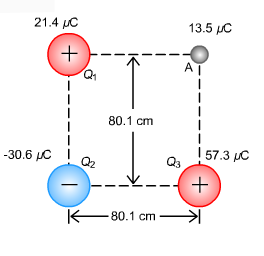# Problem: Pictured on the right are three-point charges Q1 = 21.4 μC, Q2 = -30.6 μC, and Q3 = 57.3 μC arranged according to the figure on the right. A fourth point charge is located at point A with a charge of QA = 13.5 μC. Calculate the magnitude of the net force on the charge at point A. The answer should be in N.

###### FREE Expert Solution

Coulomb's law:

$\overline{){\mathbf{F}}{\mathbf{=}}\frac{\mathbf{k}{\mathbf{q}}_{\mathbf{1}}{\mathbf{q}}_{\mathbf{2}}}{{\mathbf{r}}^{\mathbf{2}}}}$, where k is the coulomb's constant and r is the separation between the two charges.

The magnitude of the net force:

$\overline{){{\mathbf{F}}}_{\mathbf{n}\mathbf{e}\mathbf{t}}{\mathbf{=}}\sqrt{{{\mathbf{F}}_{\mathbf{x}}}^{\mathbf{2}}\mathbf{+}{{\mathbf{F}}_{\mathbf{y}}}^{\mathbf{2}}}}$

k = 9 × 109 N·m2/C2

The force due to charge Q1 is:

$\begin{array}{rcl}{\mathbf{F}}_{\mathbf{1}\mathbf{A}}& \mathbf{=}& \frac{\mathbf{k}{\mathbf{Q}}_{\mathbf{1}}{\mathbf{Q}}_{\mathbf{A}}}{{{\mathbf{r}}_{\mathbf{1}\mathbf{A}}}^{\mathbf{2}}}\\ & \mathbf{=}& \frac{\mathbf{\left(}\mathbf{9}\mathbf{×}{\mathbf{10}}^{\mathbf{9}}\mathbf{\right)}\mathbf{\left(}\mathbf{21}\mathbf{.}\mathbf{4}\mathbf{×}{\mathbf{10}}^{\mathbf{-}\mathbf{6}}\mathbf{\right)}\mathbf{\left(}\mathbf{13}\mathbf{.}\mathbf{5}\mathbf{×}{\mathbf{10}}^{\mathbf{-}\mathbf{6}}\mathbf{\right)}}{{\mathbf{\left(}\mathbf{80}\mathbf{.}\mathbf{1}\mathbf{×}{\mathbf{10}}^{\mathbf{-}\mathbf{2}}\mathbf{\right)}}^{\mathbf{2}}}\end{array}$

91% (358 ratings)###### Problem Details

Pictured on the right are three-point charges Q1 = 21.4 μC, Q2 = -30.6 μC, and Q= 57.3 μC arranged according to the figure on the right. A fourth point charge is located at point A with a charge of QA = 13.5 μC. Calculate the magnitude of the net force on the charge at point A. The answer should be in N.Frequently Asked Questions

What scientific concept do you need to know in order to solve this problem?

Our tutors have indicated that to solve this problem you will need to apply the Coulomb's Law (Electric Force) concept. You can view video lessons to learn Coulomb's Law (Electric Force). Or if you need more Coulomb's Law (Electric Force) practice, you can also practice Coulomb's Law (Electric Force) practice problems.